# Rotation

## Determining new coordinates using a picture

You can determine the new coordinates of an object

Only if
the origin is (0,0)
and only if
you rotate 90^@, 180^@ or 270^@

Remember this picture: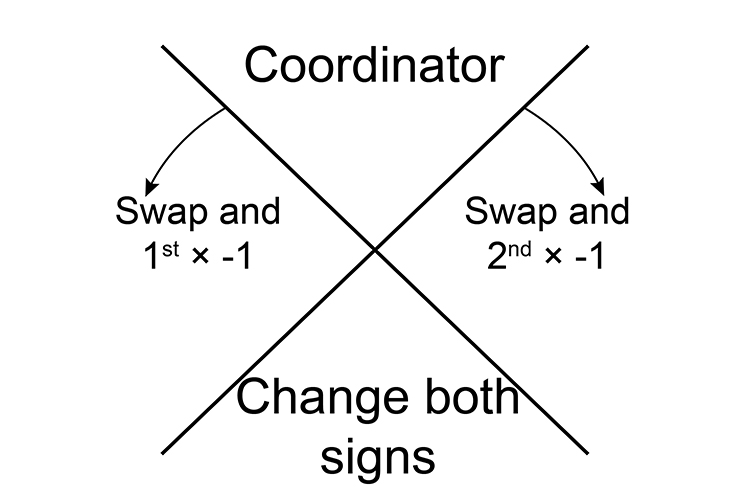The above says:

1. Move clockwise 90°
1. Swap the coordinates
2. Then multiply the second coordinate by -1
2. Move anticlockwise 90°
1. Swap the coordinates
2. Then multiply the first coordinate by -1
3. Move 180°
1. Just change both signs

Example 1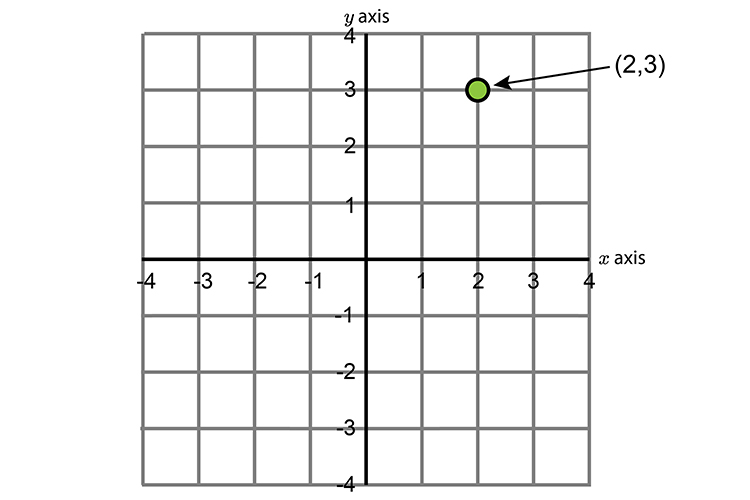With origin (0,0) rotate the point (2,3) by

1. Clockwise 90°
2. Anticlockwise 90°
3. Through 180°

To remember how to do this write out the following:1. Clockwise 90°
1. Swap the coordinates:
The coordinates are(2,3)
swap and they become (3,2)
2. Then multiply the second coordinate by -1:
(3,2)  becomes (3,-2)
2. Anticlockwise 90°
1. Swap the coordinates:
The coordinates are(2,3)
swap and they become (3,2)
2. Then multiply the first coordinate by -1:
(3,2)  becomes (-3,2)
3. Through 180°
1. Just change the signs:
The coordinates are (2,3)
(2,3)  becomes (-2,-3)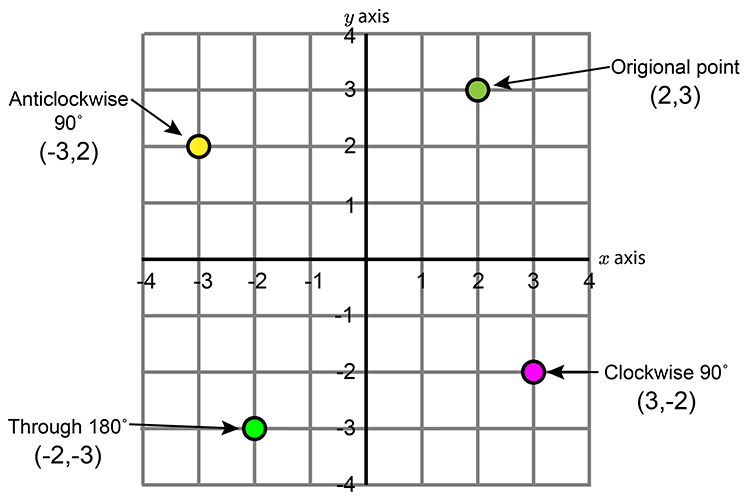Example 2

Rotate The object below anticlockwise by 90° with origin (0,0).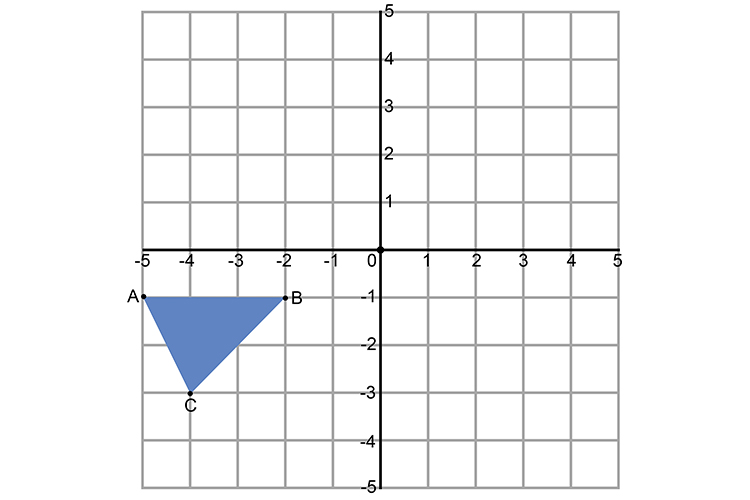So we would use:    SWAP and 1st number times-1

Point A =(-5,-1)  becomes (-1(times-1),-5)=(1,-5)

Point B =(-2,-1)  becomes (-1(times-1),-2)=(1,-2)

Point C =(-4,-3)  becomes (-3(times-1),-4)=(3,-4)

The graph becomes: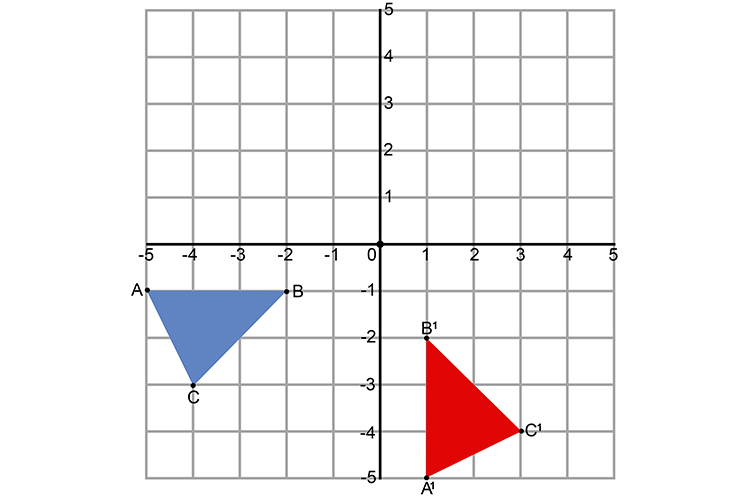Does this look correct? YES IT DOES

Example 3

Rotate the triangle below anticlockwise by 90° with origin (0,0).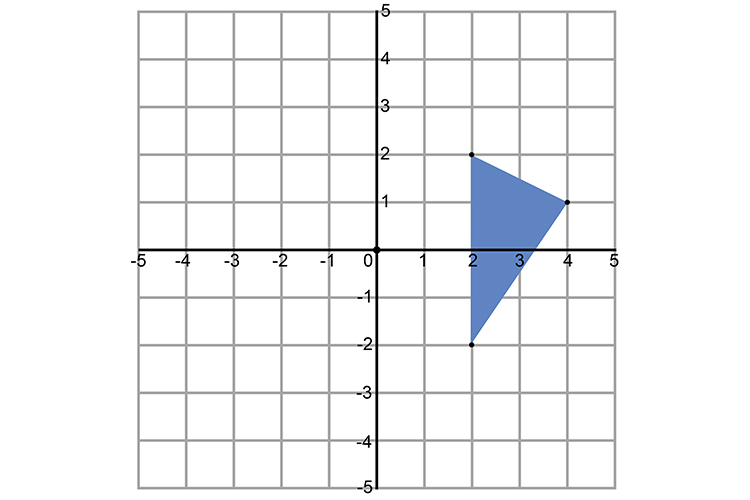UseWe would use:    SWAP and 1st number times-1

A=(1,2)  becomes (2times(-1),1)=(-2,1)

B=(3,1)  becomes (1times(-1),3)=(-1,3)

C=(2,-2)  becomes (-2times(-1),2)=(2,2)

The graph becomes: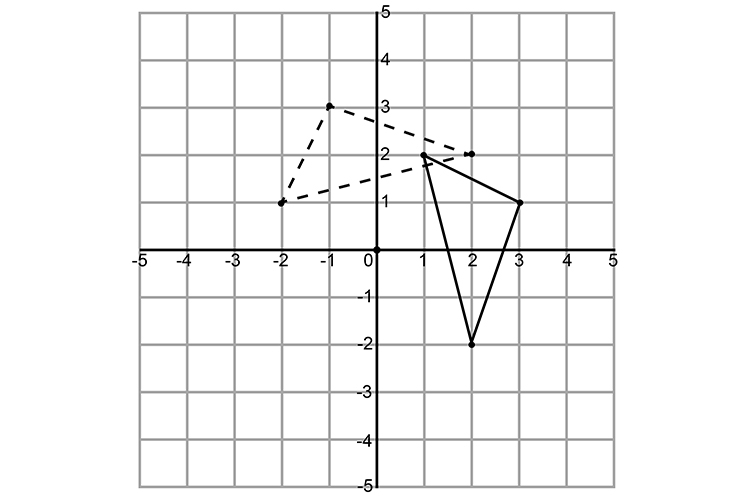Example 4

Rotate the line below by 180° with the origin at (0,0).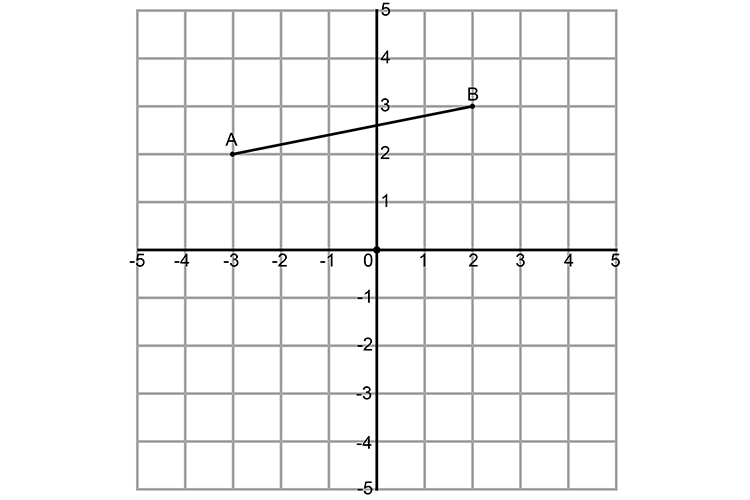UseWe would use:    Change both signs

So if A=(-3,2)  and B=(2,3)

The new coordinates would be:

A^1=(3,-2)  and B^1=(-2,-3)

Plot the results: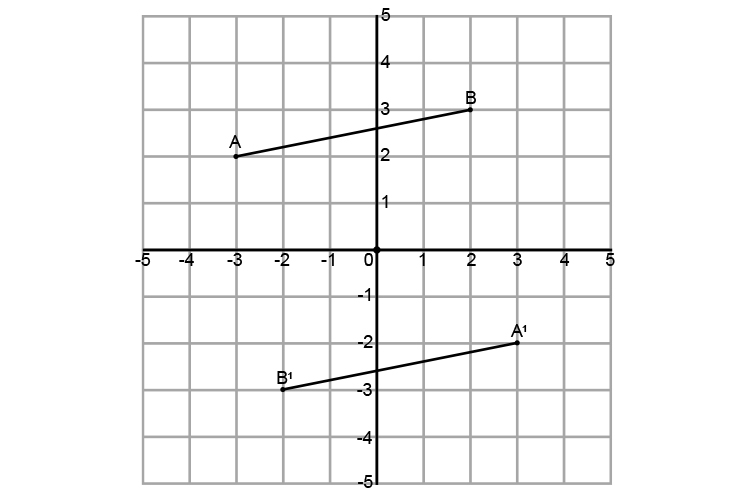As a check you could run A  and B  through the origin and see if they hitA^1  and B^1  at the same distance from the origin: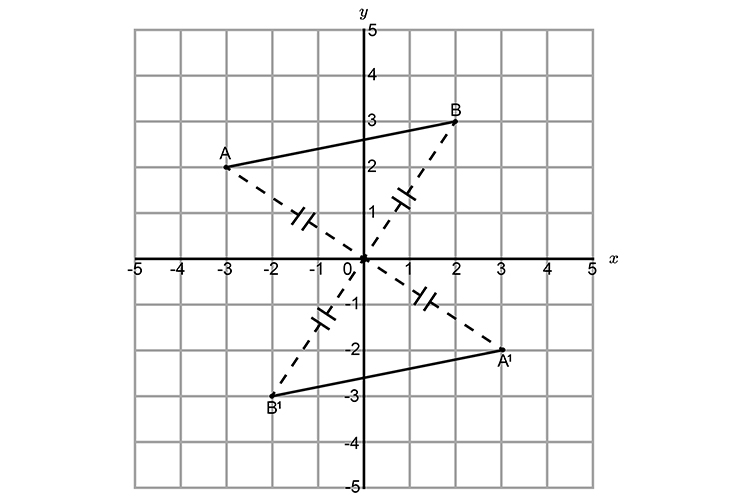This is correct.